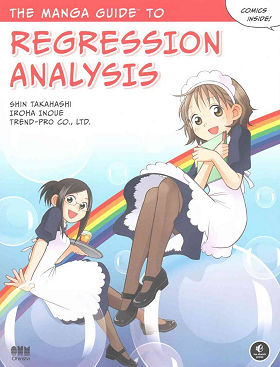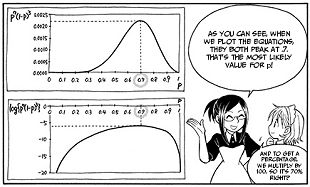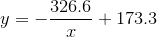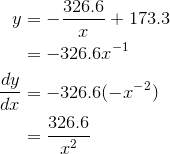Search IntMath
Close

450+ Math Lessons written by Math Professors and Teachers

5 Million+ Students Helped Each Year

1200+ Articles Written by Math Educators and Enthusiasts

Simplifying and Teaching Math for Over 23 Years

# Review: Manga Guide to Regression Analysis

By Murray Bourne, 03 Sep 2016Risa (left) and Miu on the cover of The Manga Guide to Regression Analysis

A recent addition to the "Manga Guide" series is The Manga Guide to Regression Analysis.

Each chapter in the book uses the same basic template as the others in the series:

• Manga section where one of the characters (Miu, in this case) is struggling with the math concepts, another is an expert (Risa), and a third (Hiroto) is a love interest for the struggling student. The manga section usually serves to provide a context where the mathematics will be applied. In the present book, it's a tea house and the problems solved include predicting the number of iced tea orders for a given day at a particular temperature.
• Math section which gives more rigorous explanations or background to the concepts covered in the chapter (which usually looks a lot like a normal text book).

Rinse and repeat for subsequent chapters.

Here's a typical frame from one of the manga sections:The format works quite well, since the message is made more palatable by the use of a story line (manga is always more entertaining than your average math text book), and we also get to see why it's worth our while to learn the concepts.

## Strengths

1. The concept: The Manga Guides are somewhat of a hybrid between a "serious" math text book, and a conceptual presentation of the ideas. This is certainly one of the key strengths of the series, but I would like to see them concentrate more on the latter (helping students to better grasp what is going on) and leave the heavy algebra and calculations to conventional text books (and/or to computers).

2. Clear diagrams: There are plenty of good clear diagrams throughout the book which help to develop conceptual ideas (e.g. the Correlation Coefficient diagrams on p114).

3. Clear steps: Most chapters have clearly laid out steps for finding key statistics (e.g. the Likelihood Ratio test on p179).

4. Good advice: There is good general advice here and there (e.g. on p190 where it says:

"... When you do statistics in the real world, don't just dive in and apply tests. Think about your data and purpose of the test. Without context, the numbers are just numbers and signify nothing."

5. Female role model: One pleasing point in this edition of The Manga Guides is the female role model. Risa, the "expert" in the current book, is confident and supportive of her protégé Miu.

## Gripes

While not explicitly stated, it's clear the Manga Guides are aimed at students who find math difficult and/or boring. They generally do a good job at addressing both issues, but in some places there are strange ways of explaining things.

For example:

1. Differential calculus: Starting on page 4, there is an example regarding the rate of change in Miu's height. The end game in this example is an expression for the derivative of the growth function. After plotting her height against time, an equation magically appears describing the growth (with the usual cop-out of "Don't worry where that came from - we'll see how to do it later." - but there is no reference to it later in the book).Too often in math education things "appear magically". I imagine those who are weak at algebra would not have been convinced about what followed.

In the early revision section, the differential calculus part is done numerically first using the interval of one year, then 6 months, then it jumps to "an extremely short period of time", with the analysis containing the usual mysteries of Δ (Delta) suddenly becoming zero.

Eventually we move on to algebraically differentiating the growth function, also using first principles (aka Delta method).

But I'm left wondering, "Why?". There's several pages of algebra for something that can be achieved easily using ordinary differentiation rules (as follows), which does not make a lot of sense. (In an introduction to differentiation, this would be fine, but this is a statistics book!)When they actually need to use differentiation later (e.g. on p.163 where we need the derivative of a logarithm), they (sensibly) just use the differentiation rules (not first principles). So why confuse readers unnecessarily, especially early in the book?

2. Nonlinear regression: I felt the section on nonlinear regression (p103) was very limited. Four equations are given as possible models to use, and advice is given to draw a scatter plot of the data, then match that data to one of the given functions. However, none of the mentioned functions is plotted and if students don't know what those functions look like (most won't), how will they match them with data?

There's also some discussion about plotting residuals and drawing conclusions from the shape of such plots. But there are no examples, and no plots.

This is something that a book like this could do well, but doesn't in this case. That is, they should concentrate on illustrating the main concepts (such as curve shapes and what everything means) rather than the formulas and the calculations (which should be done with computers anyway).

3. Use of computers: There's a struggle in the book regarding computer use. At times Risa (the "expert") insists Miu works everything out on paper (which would be tedious and take considerable time, and run the very real risk of the student's losing sight of the forest for the trees) and at other times she "gives in" and reaches for her laptop to solve some nasty equation.

I feel they should encourage computer use all the way and not get bogged down in endless equation solving (e.g. pp 74 & 75, which has one eye-watering step after another). What real statisticians crunch data using pen and paper? There could always be a series of appendices containing the gory details of the calculations (both the steps on paper, and how to achieve the same thing on computer) for the "interested reader".

There is an Appendix containing "Regression Calculations with Excel". This is fine, but such computer use should have been assumed throughout the book!

## Conclusion

The Manga Guides are a great way to introduce mathematical concepts to an audience that usually finds mathematics dull or something to be feared.

Similar to what I said about the Manga Guide to Linear Algebra, I felt there was an over-emphasis on calculation at the expense of the main potential of such books to explain the overall concepts. Having said that, the current volume is certainly worth a look, and I would encourage math teachers to use one or more of the good approaches illustrated by this book (base what you're doing on a story, make it clear how the math is used, use plenty of diagrams, and use female expert mathematical role models).

Disclaimers

• Images courtesy of No Starch Press
• No Starch Press sent me a review copy of The Manga Guide to Regression Analysis.
• IntMath has no commerical interest in this publication.

### 3 Comments on “Review: Manga Guide to Regression Analysis”

1. cool math says:

I like this series of books mixing manga histories with math to engage the kids and understand the concepts. Thats Great!

2. Mr Henderson says:

We are Primary pupils from the UK blogging about maths every day - we love the idea of manga maths, although regression analysis is a little complex for us!

Are there any simpler manga maths concepts?

3. Murray says:

Hello. Perhaps these ones (which are by a different author) could be better suited for you: Lost Key.

### Comment Preview

HTML: You can use simple tags like <b>, <a href="...">, etc.

To enter math, you can can either:

1. Use simple calculator-like input in the following format (surround your math in backticks, or qq on tablet or phone):
a^2 = sqrt(b^2 + c^2)
(See more on ASCIIMath syntax); or
2. Use simple LaTeX in the following format. Surround your math with $$ and $$.
$$\int g dx = \sqrt{\frac{a}{b}}$$
(This is standard simple LaTeX.)

NOTE: You can mix both types of math entry in your comment.